# Uninformed Search Methods Search Algorithms Uninformed Blind search

• Slides: 62Uninformed Search Methods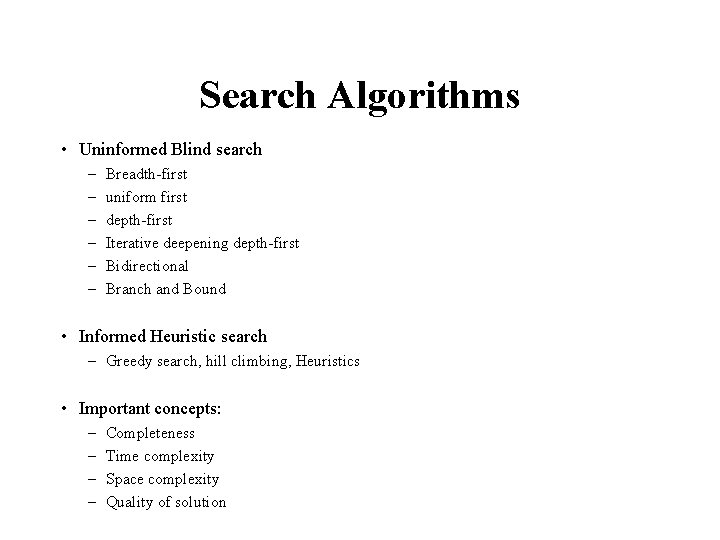Search Algorithms • Uninformed Blind search – – – Breadth-first uniform first depth-first Iterative deepening depth-first Bidirectional Branch and Bound • Informed Heuristic search – Greedy search, hill climbing, Heuristics • Important concepts: – – Completeness Time complexity Space complexity Quality of solution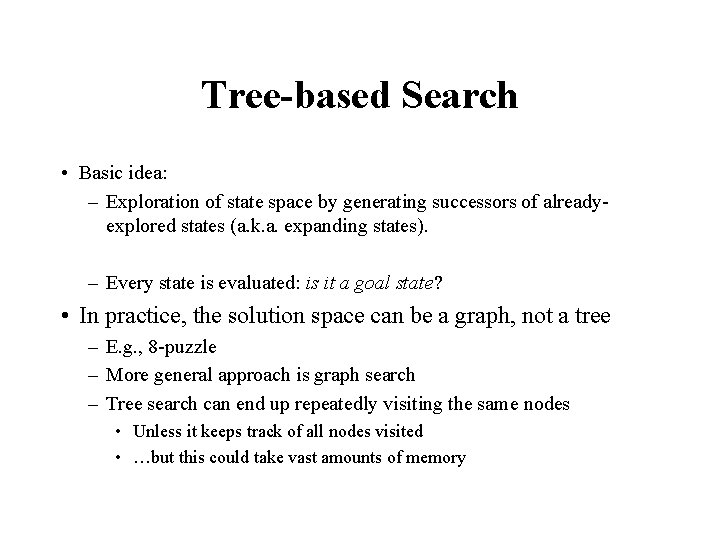Tree-based Search • Basic idea: – Exploration of state space by generating successors of alreadyexplored states (a. k. a. expanding states). – Every state is evaluated: is it a goal state? • In practice, the solution space can be a graph, not a tree – E. g. , 8 -puzzle – More general approach is graph search – Tree search can end up repeatedly visiting the same nodes • Unless it keeps track of all nodes visited • …but this could take vast amounts of memory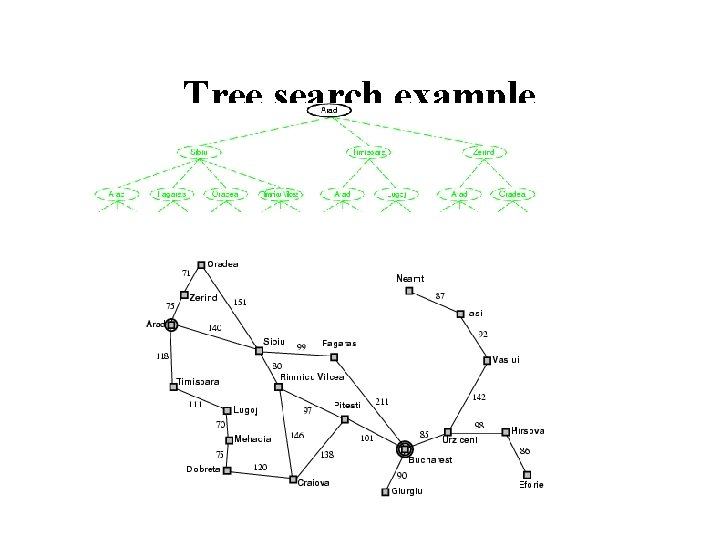Tree search exampleTree search example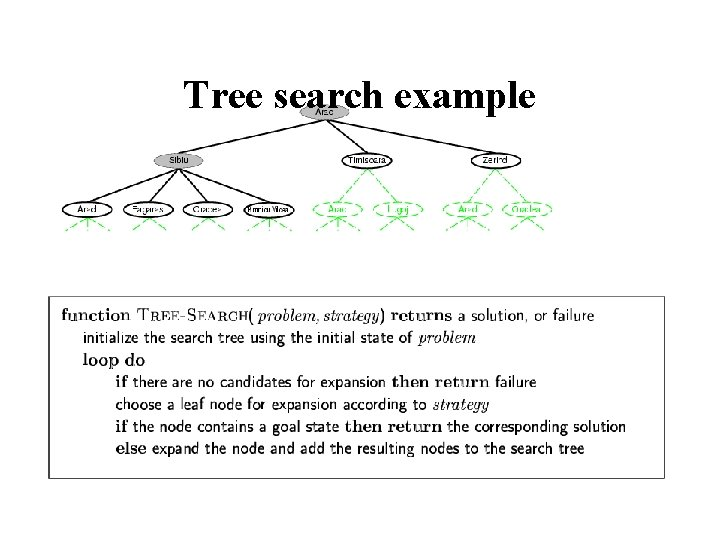Tree search exampleTree search example This “strategy” is what differentiates different search algorithmsStates versus Nodes • A state is a (representation of) a physical configuration • A node is a data structure constituting part of a search tree contains info such as: state, parent node, action, path cost g(x), depth • The Expand function creates new nodes, filling in the various fields and using the Successor. Fn of the problem to create the corresponding states.Search Tree for the 8 puzzle problem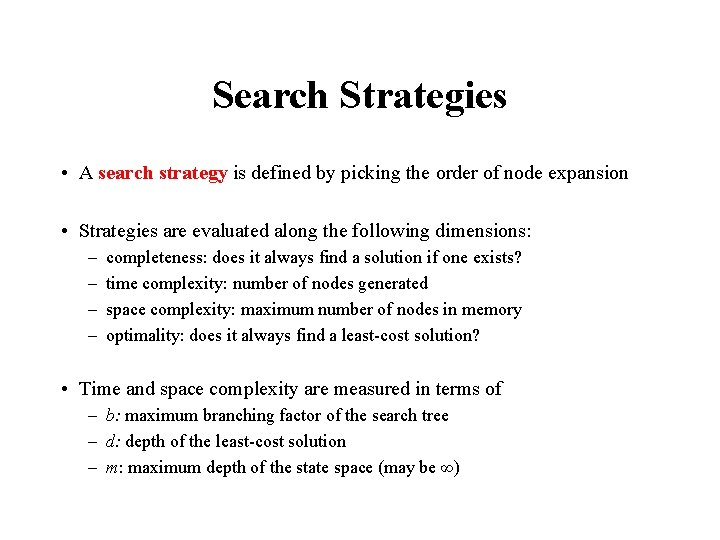Search Strategies • A search strategy is defined by picking the order of node expansion • Strategies are evaluated along the following dimensions: – – completeness: does it always find a solution if one exists? time complexity: number of nodes generated space complexity: maximum number of nodes in memory optimality: does it always find a least-cost solution? • Time and space complexity are measured in terms of – b: maximum branching factor of the search tree – d: depth of the least-cost solution – m: maximum depth of the state space (may be ∞)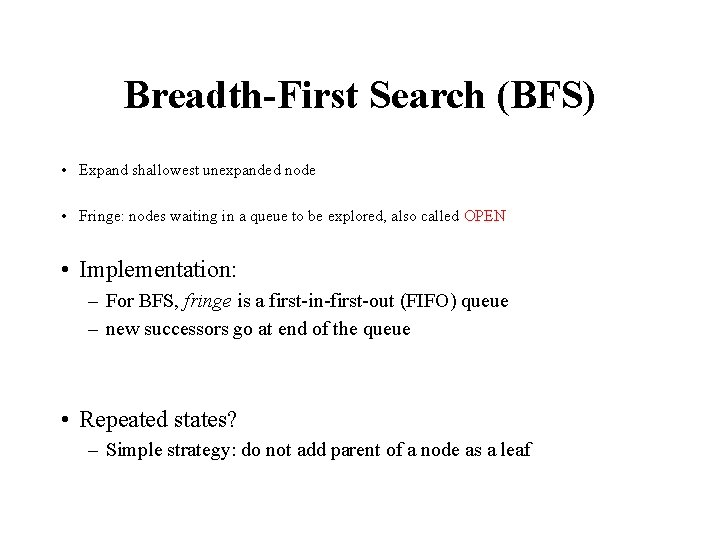Breadth-First Search (BFS) • Expand shallowest unexpanded node • Fringe: nodes waiting in a queue to be explored, also called OPEN • Implementation: – For BFS, fringe is a first-in-first-out (FIFO) queue – new successors go at end of the queue • Repeated states? – Simple strategy: do not add parent of a node as a leaf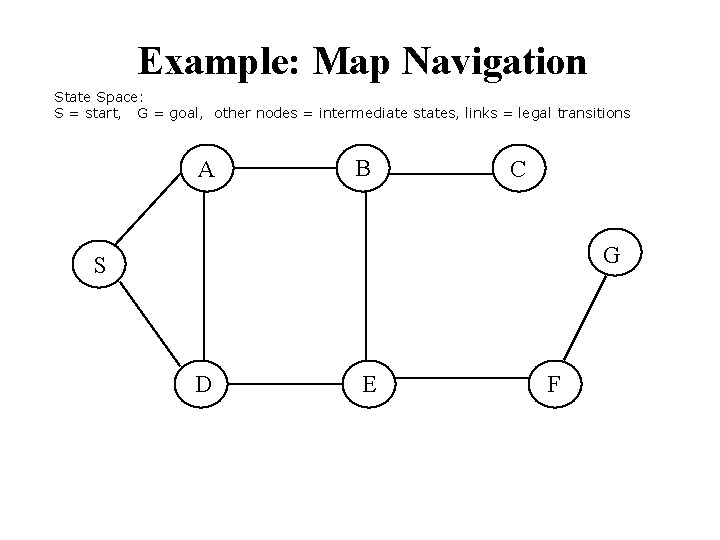Example: Map Navigation State Space: S = start, G = goal, other nodes = intermediate states, links = legal transitions A B C G S D E FBFS Search Tree A S B C G S D E Queue = {S} Select S Goal(S) = true? If not, Expand(S) F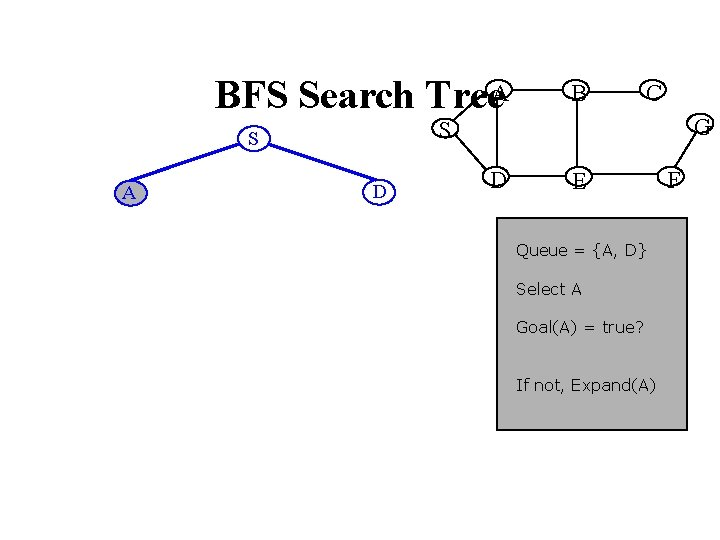BFS Search Tree. A C G S S A B D D E Queue = {A, D} Select A Goal(A) = true? If not, Expand(A) FBFS Search Tree. A B D D C G S S A B D E F Queue = {D, B, D} Select D Goal(D) = true? If not, expand(D)BFS Search Tree. A D D A D C G S S B B A E E F Queue = {B, D, A, E} Select B etc.BFS Search Tree. A D D A C A D E S E C G S S B B S E F E B B F Level 3 Queue = {C, E, S, B, B, F}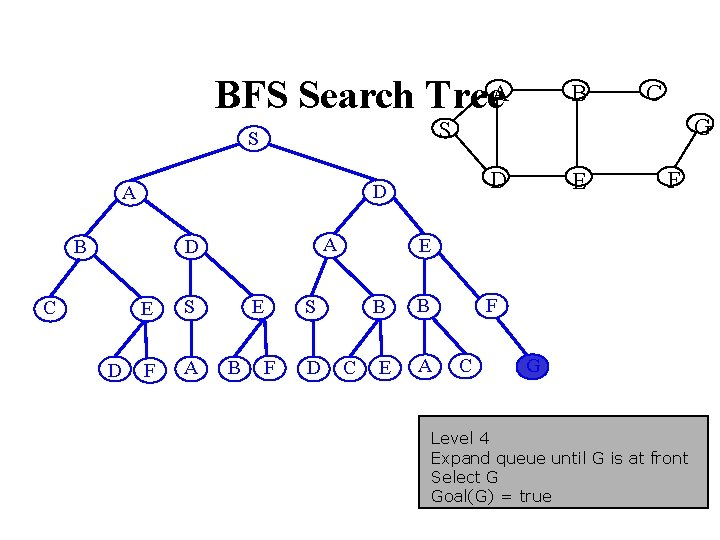BFS Search Tree. A A D D E S F A E B G D D A C C S S B B F F E S D E C B B F E A C G Level 4 Expand queue until G is at front Select G Goal(G) = trueDepth-First Search (BFS) • Expand deepest unexpanded node • Implementation: – For DFS, fringe is a first-in-first-out (FIFO) queue – new successors go at beginning of the queue • Repeated nodes? – Simple strategy: Do not add a state as a leaf if that state is on the path from the root to the current nodeDFS Search Tree A C G S S A B D D E F Queue = {A, D}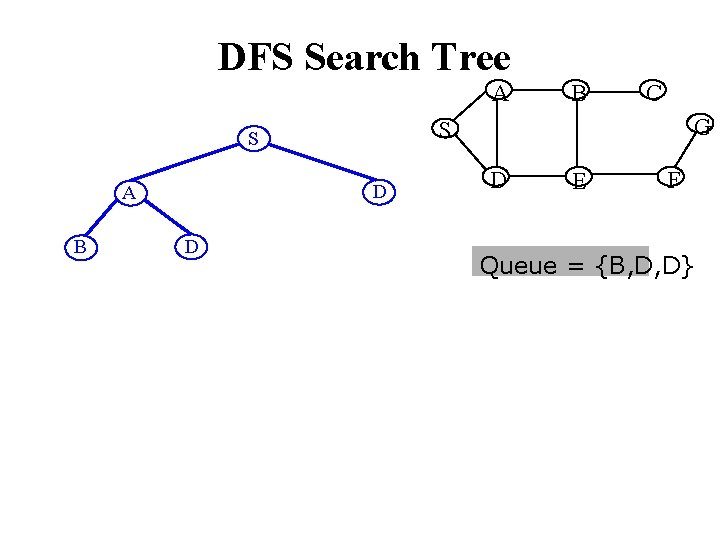DFS Search Tree A B D D C G S S A B D E F Queue = {B, D, D}DFS Search Tree. A D B C D E C G S S A B D E F Queue = {C, E, D, D}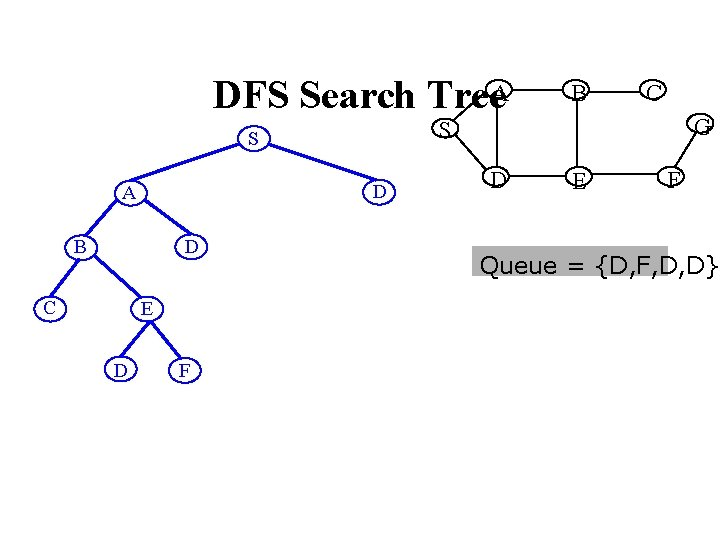DFS Search Tree. A D B D C E D F C G S S A B D E F Queue = {D, F, D, D}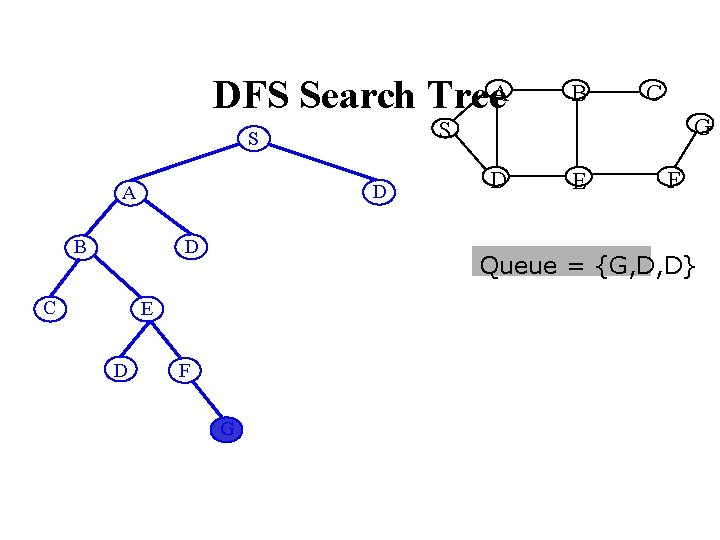DFS Search Tree. A D B D C G D E F Queue = {G, D, D} E D C S S A B F G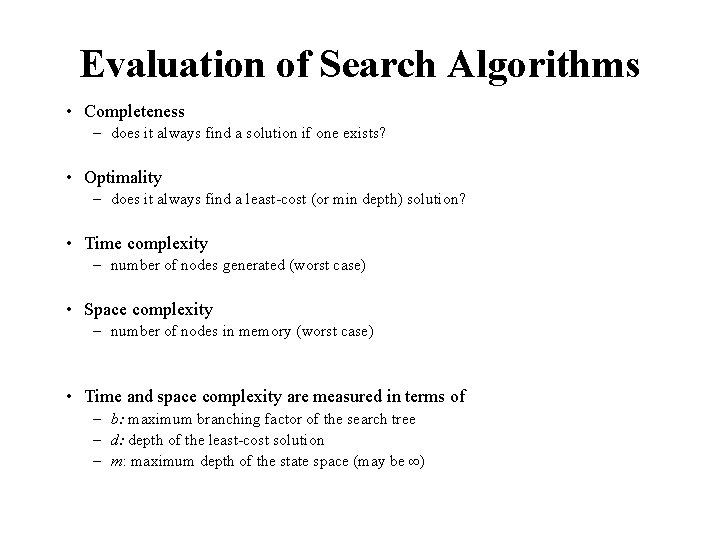Evaluation of Search Algorithms • Completeness – does it always find a solution if one exists? • Optimality – does it always find a least-cost (or min depth) solution? • Time complexity – number of nodes generated (worst case) • Space complexity – number of nodes in memory (worst case) • Time and space complexity are measured in terms of – b: maximum branching factor of the search tree – d: depth of the least-cost solution – m: maximum depth of the state space (may be ∞)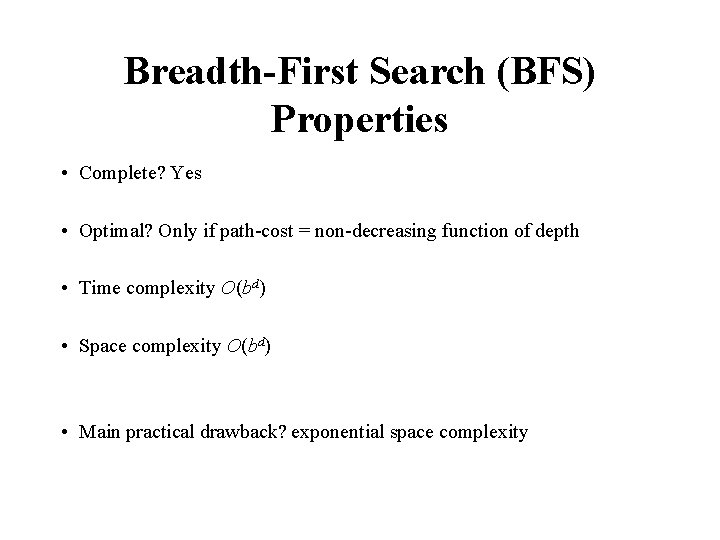Breadth-First Search (BFS) Properties • Complete? Yes • Optimal? Only if path-cost = non-decreasing function of depth • Time complexity O(bd) • Space complexity O(bd) • Main practical drawback? exponential space complexity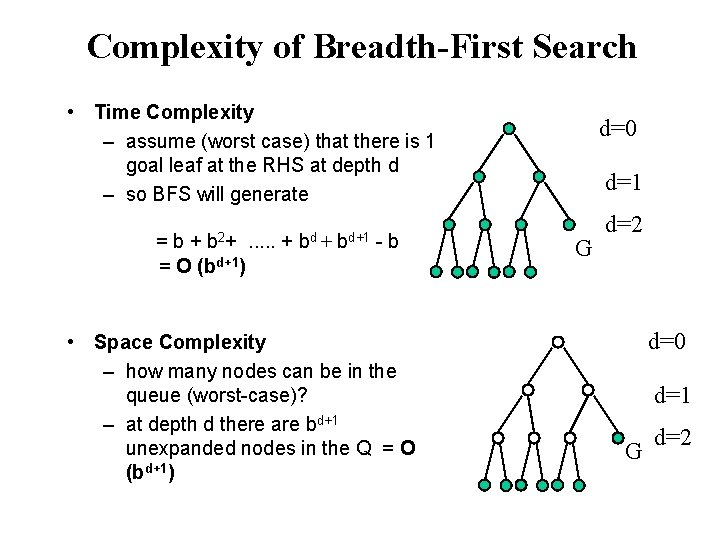Complexity of Breadth-First Search • Time Complexity – assume (worst case) that there is 1 goal leaf at the RHS at depth d – so BFS will generate b 2+ =b+. . . + = O (bd+1) bd + bd+1 -b • Space Complexity – how many nodes can be in the queue (worst-case)? – at depth d there are bd+1 unexpanded nodes in the Q = O (bd+1) d=0 d=1 G d=2Examples of Time and Memory Requirements for Breadth-First Search Assuming b=10, 10000 nodes/sec, 1 kbyte/node Depth of Solution Nodes Generated Time Memory 2 1100 0. 11 seconds 1 MB 4 111, 100 11 seconds 106 MB 8 109 31 hours 1 TB 12 1013 35 years 10 PB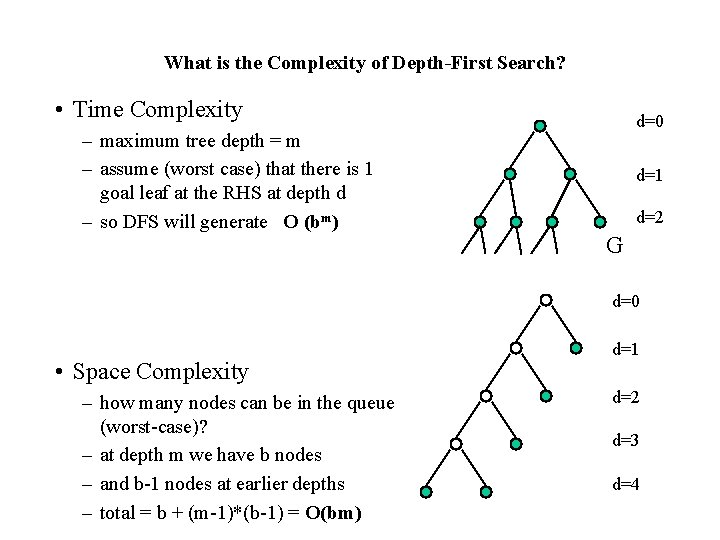What is the Complexity of Depth-First Search? • Time Complexity – maximum tree depth = m – assume (worst case) that there is 1 goal leaf at the RHS at depth d – so DFS will generate O (bm) d=0 d=1 d=2 G d=0 • Space Complexity – how many nodes can be in the queue (worst-case)? – at depth m we have b nodes – and b-1 nodes at earlier depths – total = b + (m-1)*(b-1) = O(bm) d=1 d=2 d=3 d=4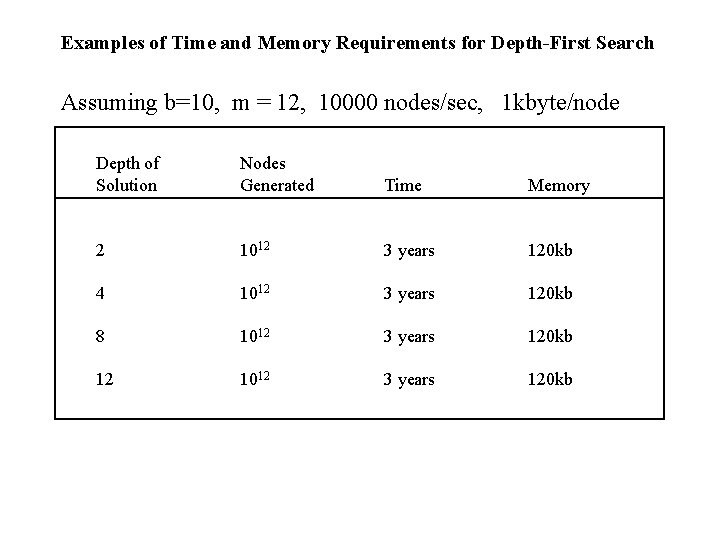Examples of Time and Memory Requirements for Depth-First Search Assuming b=10, m = 12, 10000 nodes/sec, 1 kbyte/node Depth of Solution Nodes Generated Time Memory 2 1012 3 years 120 kb 4 1012 3 years 120 kb 8 1012 3 years 120 kb 12 1012 3 years 120 kb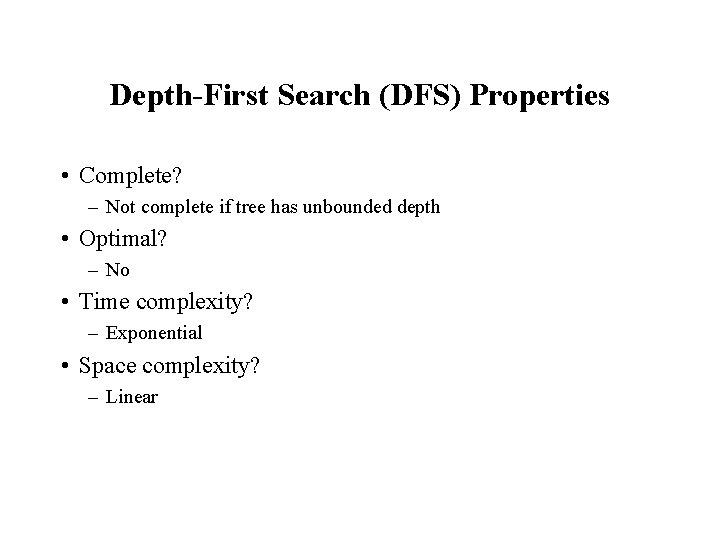Depth-First Search (DFS) Properties • Complete? – Not complete if tree has unbounded depth • Optimal? – No • Time complexity? – Exponential • Space complexity? – Linear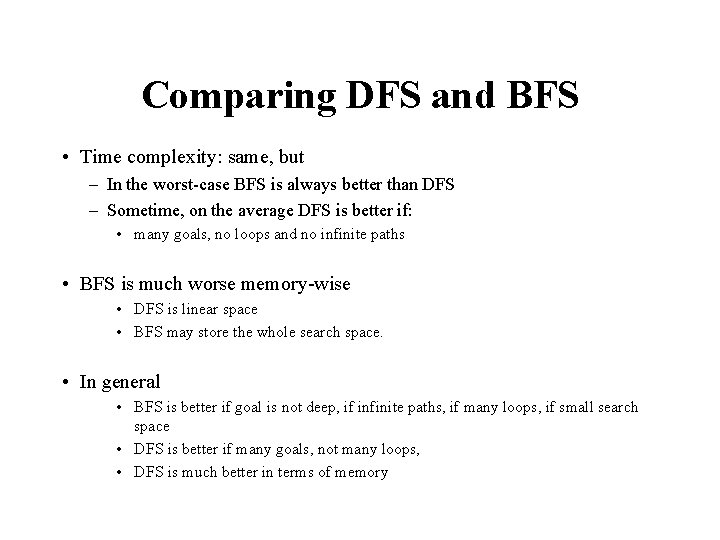Comparing DFS and BFS • Time complexity: same, but – In the worst-case BFS is always better than DFS – Sometime, on the average DFS is better if: • many goals, no loops and no infinite paths • BFS is much worse memory-wise • DFS is linear space • BFS may store the whole search space. • In general • BFS is better if goal is not deep, if infinite paths, if many loops, if small search space • DFS is better if many goals, not many loops, • DFS is much better in terms of memory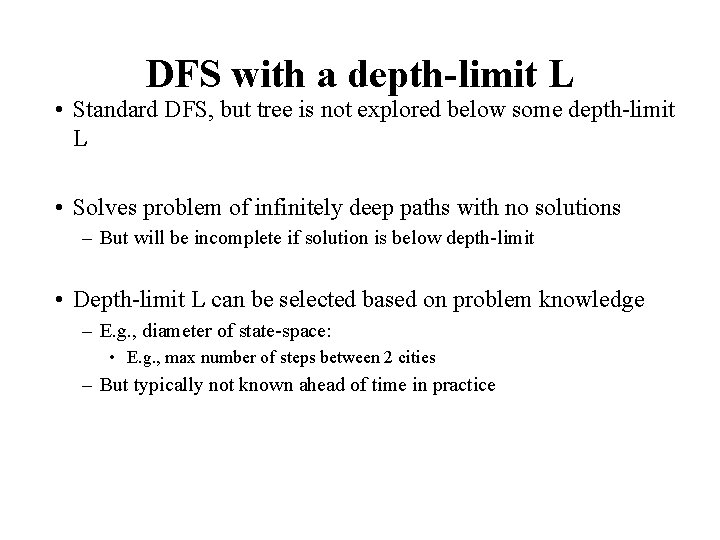DFS with a depth-limit L • Standard DFS, but tree is not explored below some depth-limit L • Solves problem of infinitely deep paths with no solutions – But will be incomplete if solution is below depth-limit • Depth-limit L can be selected based on problem knowledge – E. g. , diameter of state-space: • E. g. , max number of steps between 2 cities – But typically not known ahead of time in practice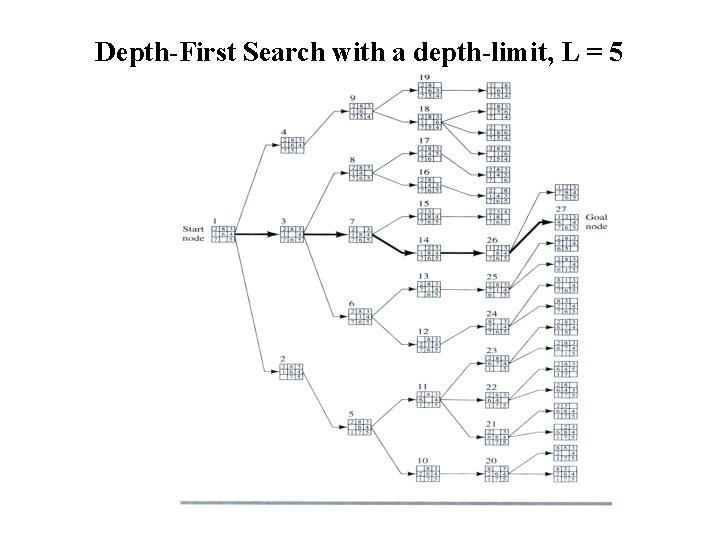Depth-First Search with a depth-limit, L = 5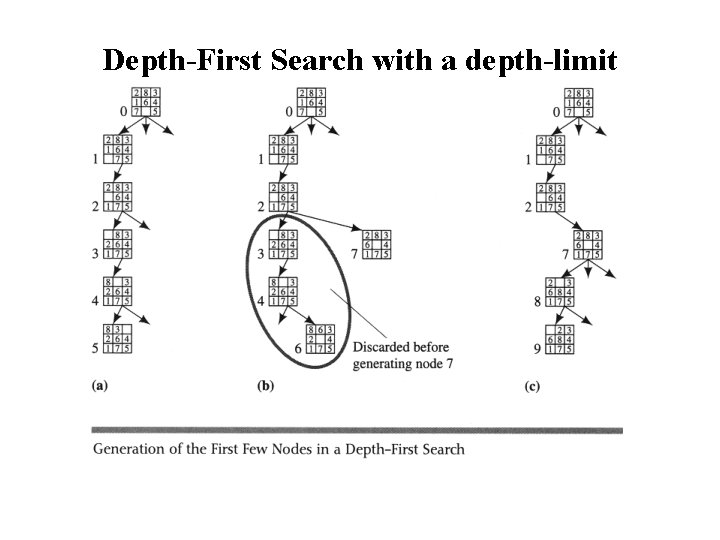Depth-First Search with a depth-limitIterative Deepening Search (IDS) • Run multiple DFS searches with increasing depth-limits Iterative deepening search ¢L = 1 ¢ While no solution, do ¢ DFS from initial state S 0 with cutoff L ¢ If found goal, ¢ stop and return solution, ¢ else, increment depth limit L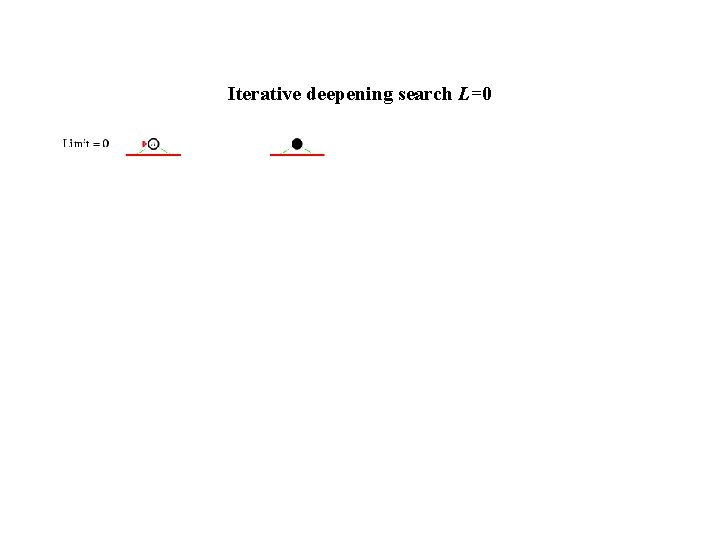Iterative deepening search L=0Iterative deepening search L=1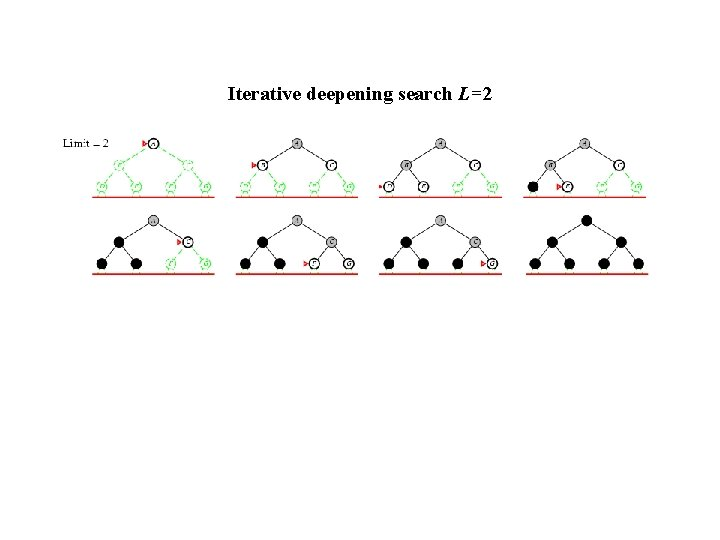Iterative deepening search L=2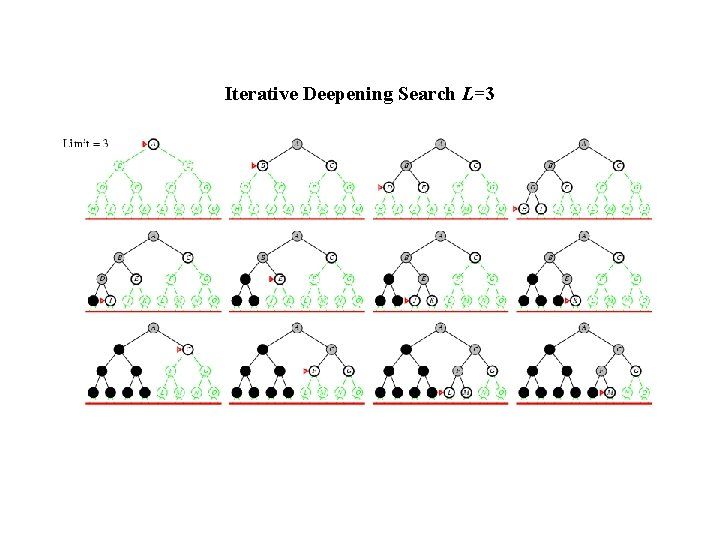Iterative Deepening Search L=3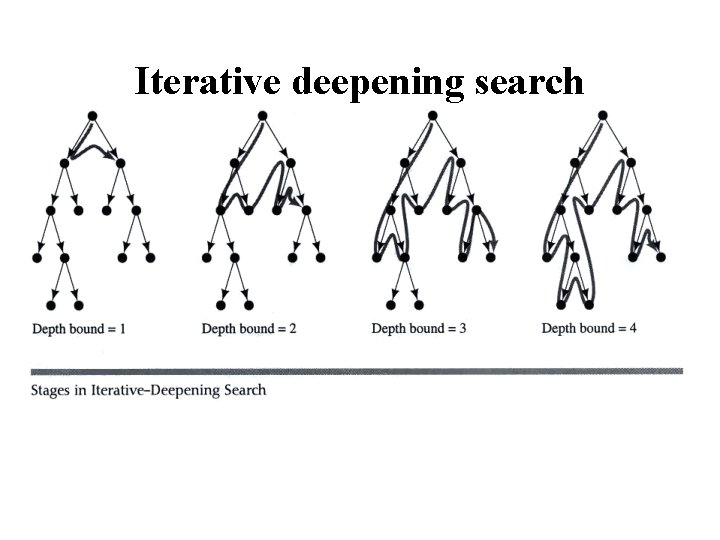Iterative deepening searchProperties of Iterative Deepening Search • Space complexity = O(bd) • (since its like depth first search run different times, with maximum depth limit d) • Time Complexity • b + (b+b 2) +. . . . (b+. . bd) = O(bd) (i. e. , asymptotically the same as BFS or DFS to limited depth d in the worst case) • Complete? – Yes • Optimal – Only if path cost is a non-decreasing function of depth • IDS combines the small memory footprint of DFS, and has the completeness guarantee of BFS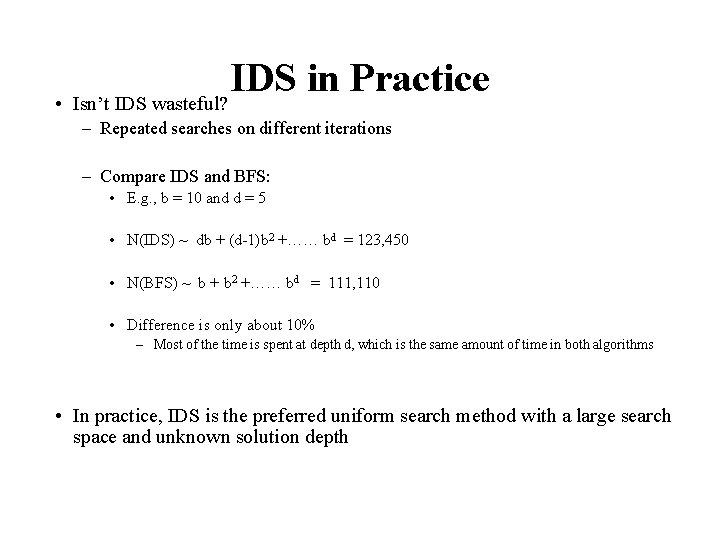IDS in Practice • Isn’t IDS wasteful? – Repeated searches on different iterations – Compare IDS and BFS: • E. g. , b = 10 and d = 5 • N(IDS) ~ db + (d-1)b 2 +…… bd = 123, 450 • N(BFS) ~ b + b 2 +…… bd = 111, 110 • Difference is only about 10% – Most of the time is spent at depth d, which is the same amount of time in both algorithms • In practice, IDS is the preferred uniform search method with a large search space and unknown solution depth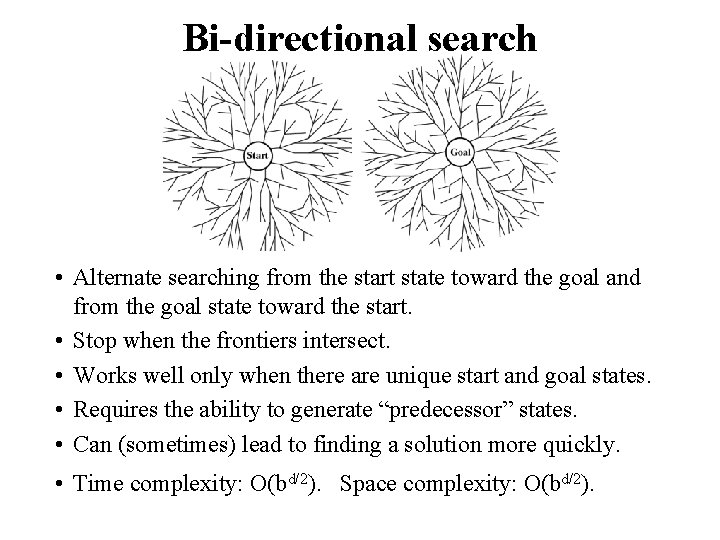Bi-directional search • Alternate searching from the start state toward the goal and from the goal state toward the start. • Stop when the frontiers intersect. • Works well only when there are unique start and goal states. • Requires the ability to generate “predecessor” states. • Can (sometimes) lead to finding a solution more quickly. • Time complexity: O(bd/2). Space complexity: O(bd/2).Bidirectional Search • Idea – simultaneously search forward from S and backwards from G – stop when both “meet in the middle” – need to keep track of the intersection of 2 open sets of nodes • What does searching backwards from G mean – need a way to specify the predecessors of G • this can be difficult, • e. g. , predecessors of checkmate in chess? – what if there are multiple goal states? – what if there is only a goal test, no explicit list? • Complexity – time complexity at best is: O(2 b(d/2)) = O(b (d/2)) – memory complexity is the same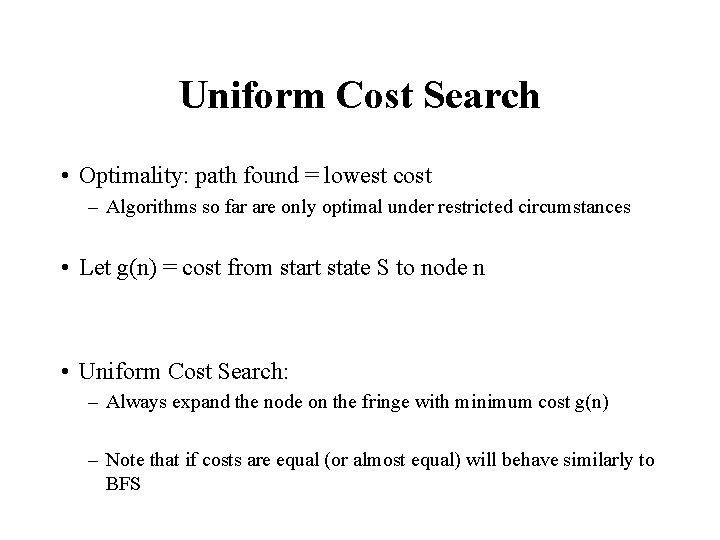Uniform Cost Search • Optimality: path found = lowest cost – Algorithms so far are only optimal under restricted circumstances • Let g(n) = cost from start state S to node n • Uniform Cost Search: – Always expand the node on the fringe with minimum cost g(n) – Note that if costs are equal (or almost equal) will behave similarly to BFSUniform Cost Search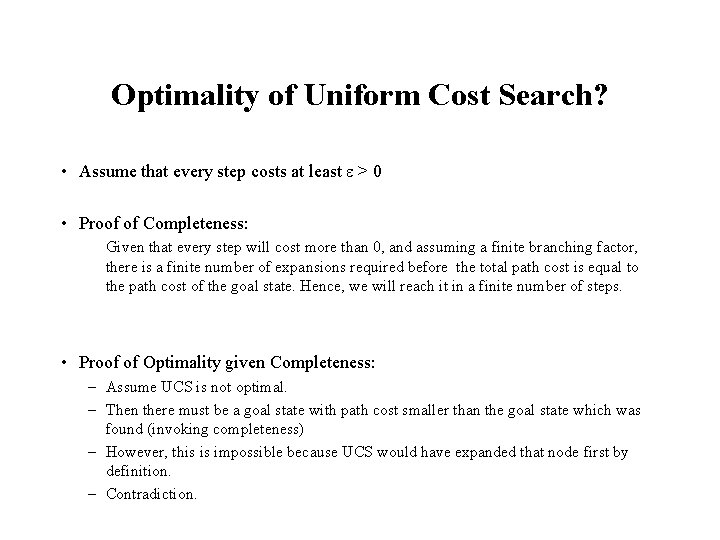Optimality of Uniform Cost Search? • Assume that every step costs at least e > 0 • Proof of Completeness: Given that every step will cost more than 0, and assuming a finite branching factor, there is a finite number of expansions required before the total path cost is equal to the path cost of the goal state. Hence, we will reach it in a finite number of steps. • Proof of Optimality given Completeness: – Assume UCS is not optimal. – Then there must be a goal state with path cost smaller than the goal state which was found (invoking completeness) – However, this is impossible because UCS would have expanded that node first by definition. – Contradiction.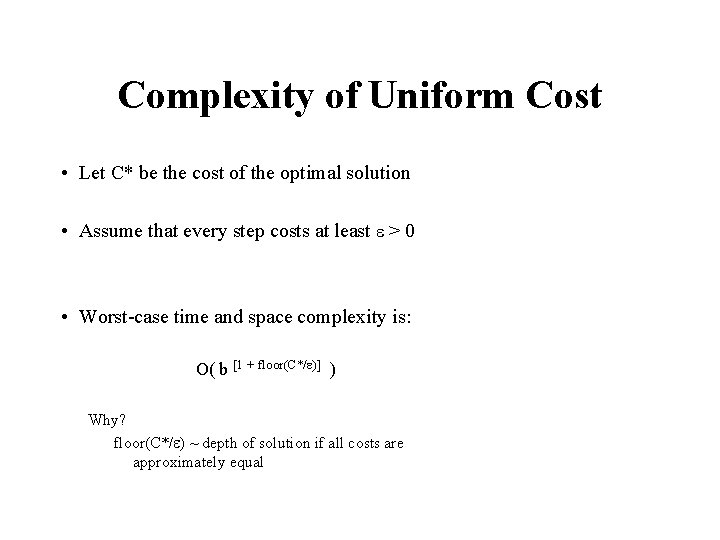Complexity of Uniform Cost • Let C* be the cost of the optimal solution • Assume that every step costs at least e > 0 • Worst-case time and space complexity is: O( b [1 + floor(C*/e)] ) Why? floor(C*/e) ~ depth of solution if all costs are approximately equalAverage case complexity of these algorithms? • How would we do an average case analysis of these algorithms? • E. g. , single goal in a tree of maximum depth m – Solution randomly located at depth d? – Solution randomly located in the search tree? – Solution randomly located in state-space? – What about multiple solutions? [left as an exercise]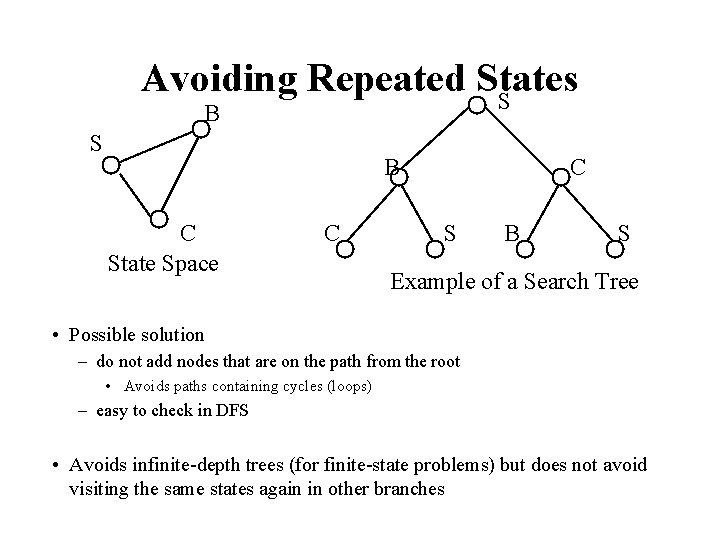Avoiding Repeated States S B C State Space C C S B S Example of a Search Tree • Possible solution – do not add nodes that are on the path from the root • Avoids paths containing cycles (loops) – easy to check in DFS • Avoids infinite-depth trees (for finite-state problems) but does not avoid visiting the same states again in other branchesRepeated States • Failure to detect repeated states can turn a linear problem into an exponential one!SummaryBreadth-First • Enqueue nodes on nodes in FIFO (first-in, first-out) order. • Complete • Optimal (i. e. , admissible) if all operators have the same cost. Otherwise, not optimal but finds solution with shortest path length. • Exponential time and space complexity, O(bd), where d is the depth of the solution and b is the branching factor (i. e. , number of children) at each node • Will take a long time to find solutions with a large number of steps because must look at all shorter length possibilities first – A complete search tree of depth d where each non-leaf node has b children, has a total of 1 + b 2 +. . . + bd = (b(d+1) - 1)/(b-1) nodes – For a complete search tree of depth 12, where every node at depths 0, . . . , 11 has 10 children and every node at depth 12 has 0 children, there are 1 + 1000 +. . . + 1012 = (1013 - 1)/9 = O(1012) nodes in the complete search tree. If BFS expands 1000 nodes/sec and each node uses 100 bytes of storage, then BFS will take 35 years to run in the worst case, and it will use 111 terabytes of memory!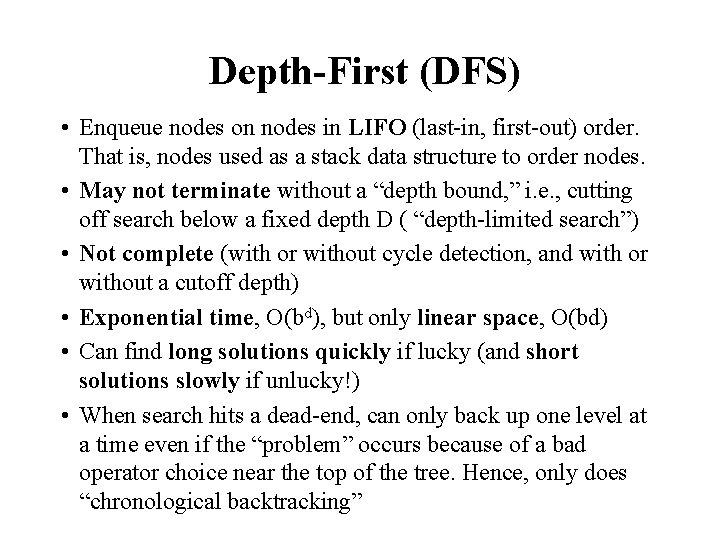Depth-First (DFS) • Enqueue nodes on nodes in LIFO (last-in, first-out) order. That is, nodes used as a stack data structure to order nodes. • May not terminate without a “depth bound, ” i. e. , cutting off search below a fixed depth D ( “depth-limited search”) • Not complete (with or without cycle detection, and with or without a cutoff depth) • Exponential time, O(bd), but only linear space, O(bd) • Can find long solutions quickly if lucky (and short solutions slowly if unlucky!) • When search hits a dead-end, can only back up one level at a time even if the “problem” occurs because of a bad operator choice near the top of the tree. Hence, only does “chronological backtracking”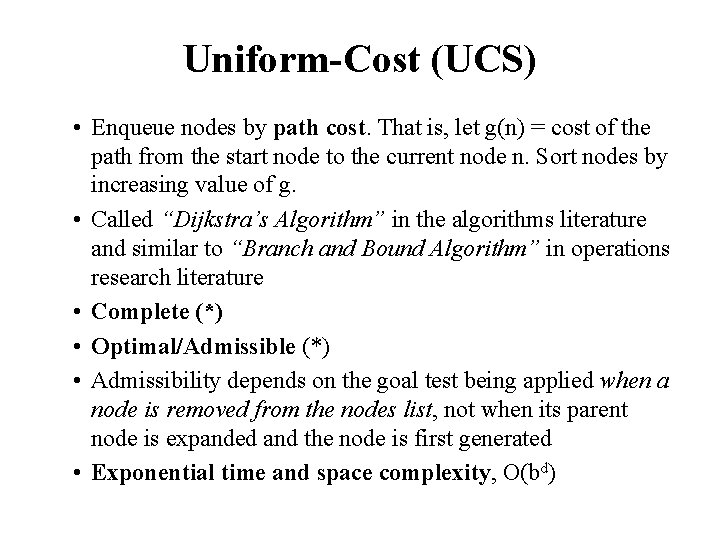Uniform-Cost (UCS) • Enqueue nodes by path cost. That is, let g(n) = cost of the path from the start node to the current node n. Sort nodes by increasing value of g. • Called “Dijkstra’s Algorithm” in the algorithms literature and similar to “Branch and Bound Algorithm” in operations research literature • Complete (*) • Optimal/Admissible (*) • Admissibility depends on the goal test being applied when a node is removed from the nodes list, not when its parent node is expanded and the node is first generated • Exponential time and space complexity, O(bd)Depth-First Iterative Deepening (DFID) • First do DFS to depth 0 (i. e. , treat start node as having no successors), then, if no solution found, do DFS to depth 1, etc. until solution found do DFS with depth cutoff c c = c+1 • Complete • Optimal/Admissible if all operators have the same cost. Otherwise, not optimal but guarantees finding solution of shortest length (like BFS). • Time complexity seems worse than BFS or DFS because nodes near the top of the search tree are generated multiple times, but because almost all of the nodes are near the bottom of a tree, the worst case time complexity is still exponential, O(bd).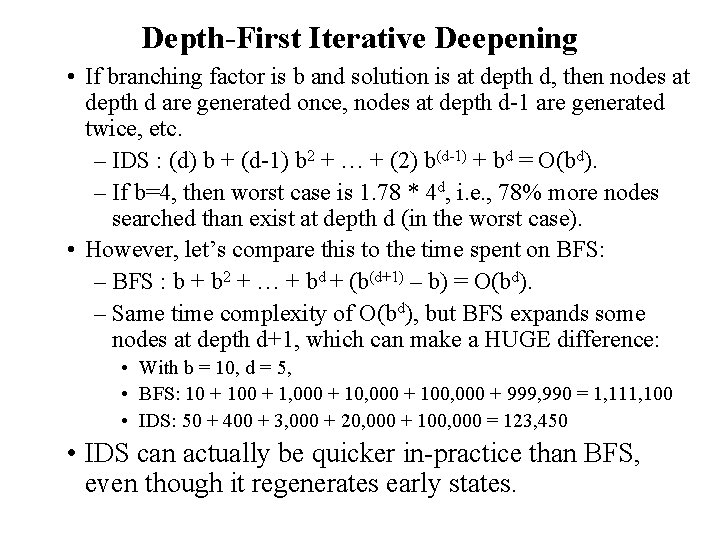Depth-First Iterative Deepening • If branching factor is b and solution is at depth d, then nodes at depth d are generated once, nodes at depth d-1 are generated twice, etc. – IDS : (d) b + (d-1) b 2 + … + (2) b(d-1) + bd = O(bd). – If b=4, then worst case is 1. 78 * 4 d, i. e. , 78% more nodes searched than exist at depth d (in the worst case). • However, let’s compare this to the time spent on BFS: – BFS : b + b 2 + … + bd + (b(d+1) – b) = O(bd). – Same time complexity of O(bd), but BFS expands some nodes at depth d+1, which can make a HUGE difference: • With b = 10, d = 5, • BFS: 10 + 100 + 1, 000 + 100, 000 + 999, 990 = 1, 111, 100 • IDS: 50 + 400 + 3, 000 + 20, 000 + 100, 000 = 123, 450 • IDS can actually be quicker in-practice than BFS, even though it regenerates early states.Depth-First Iterative Deepening • Exponential time complexity, O(bd), like BFS • Linear space complexity, O(bd), like DFS • Has advantage of BFS (i. e. , completeness) and also advantages of DFS (i. e. , limited space and finds longer paths more quickly) • Generally preferred for large state spaces where solution depth is unknown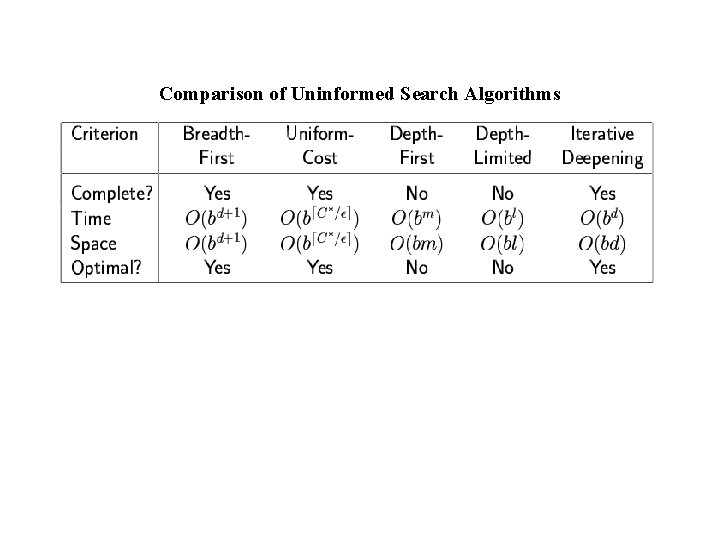Comparison of Uninformed Search Algorithms